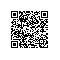# 话说模式匹配(7) 一个构造器模式的例子(by case class)

scala里的List是个典型的很适用模式匹配的结构，它的接口和数据定义非常凝练。现在我们假设需要一个与List结构正好相反的结构MyList。

MyList也由2部分组成 [init, last]，其中last是元素本身，而init则是MyList类型。(与List正好颠倒)

// 定义抽象类
abstract class MyList[+A]

// 具体子类，数据由两部分组成:init,last
case class Cons[B] (init:MyList[B], last:B) extends MyList[B]

// 元素为空的MyList单例对象，类似 Nil
case object Empty extends MyList[Nothing]


scala> val a = Cons(Empty,1)
a: Cons[Int] = Cons(Empty,1)

scala> a.last
res0: Int = 1

// 嵌套
scala> val b = Cons(Cons(Empty,1),2)
b: Cons[Int] = Cons(Cons(Empty,1),2)

// 模式匹配(Cons可以用中缀表达)
scala> b match{ case x Cons 1 Cons 2 => print(x) }
Empty


object MyList {
def apply[A](xs:A*):MyList[A] = {
var r:MyList[A] = null
var t:MyList[A] = Empty
for(x<-xs) { r = Cons(t,x); t=r}
r
}
}


eg：

scala> MyList(1,2,3) match{ case x Cons 2 Cons 3 => print(x)}
Cons(Empty,1)

scala> MyList(1,2,3,4) match{ case x Cons 4 => print(x) }
Cons(Cons(Cons(Empty,1),2),3)

文章转自 并发编程网-ifeve.com使用钉钉扫一扫加入圈子
+ 订阅